Check Important Questions and Chapter Summary -https://you.tube/Real-Numbers-Class-10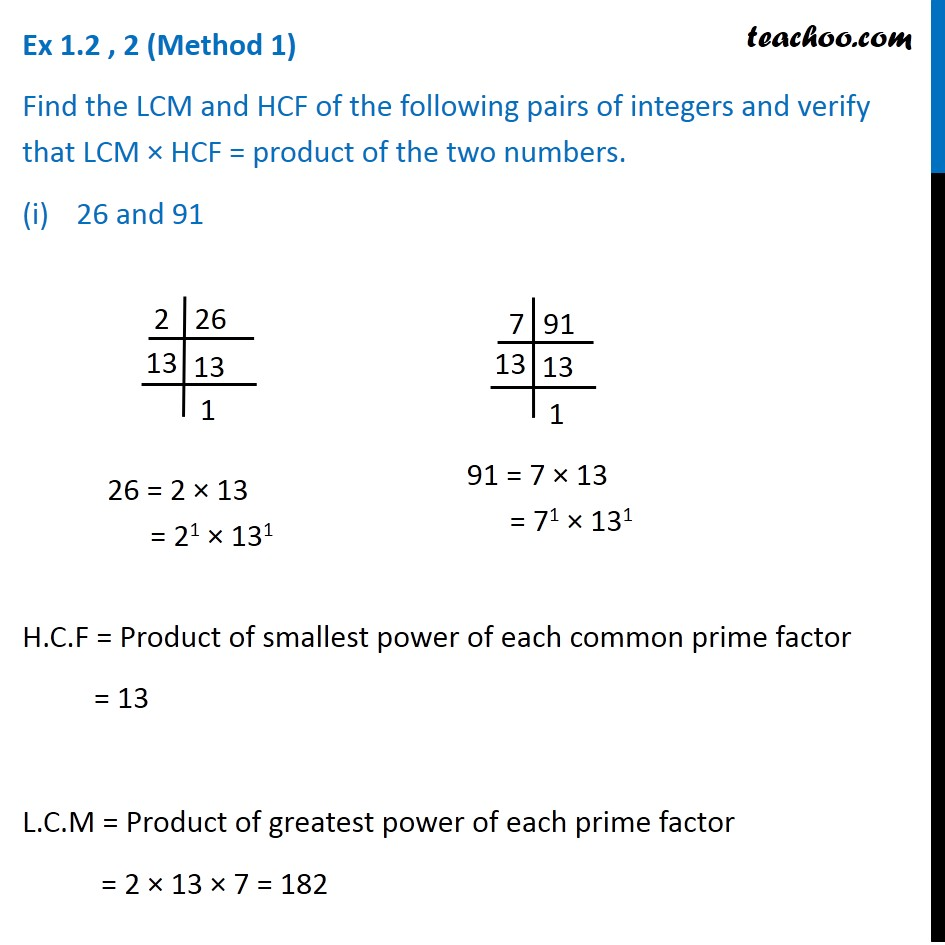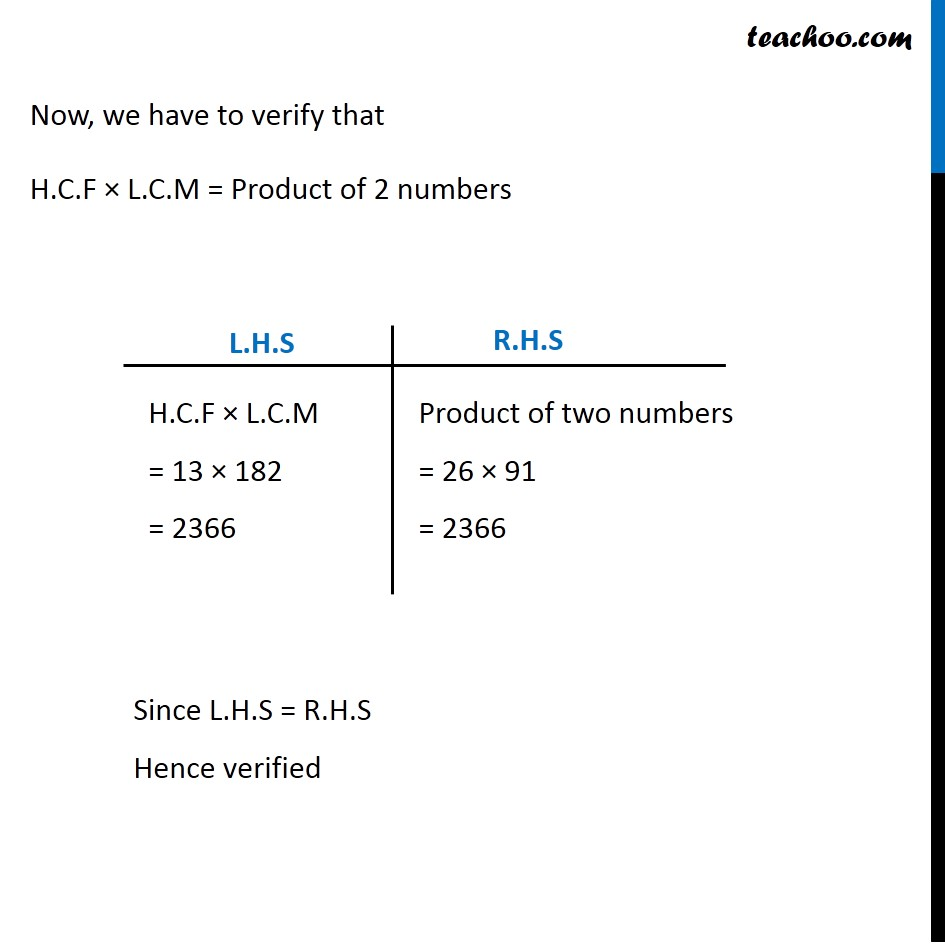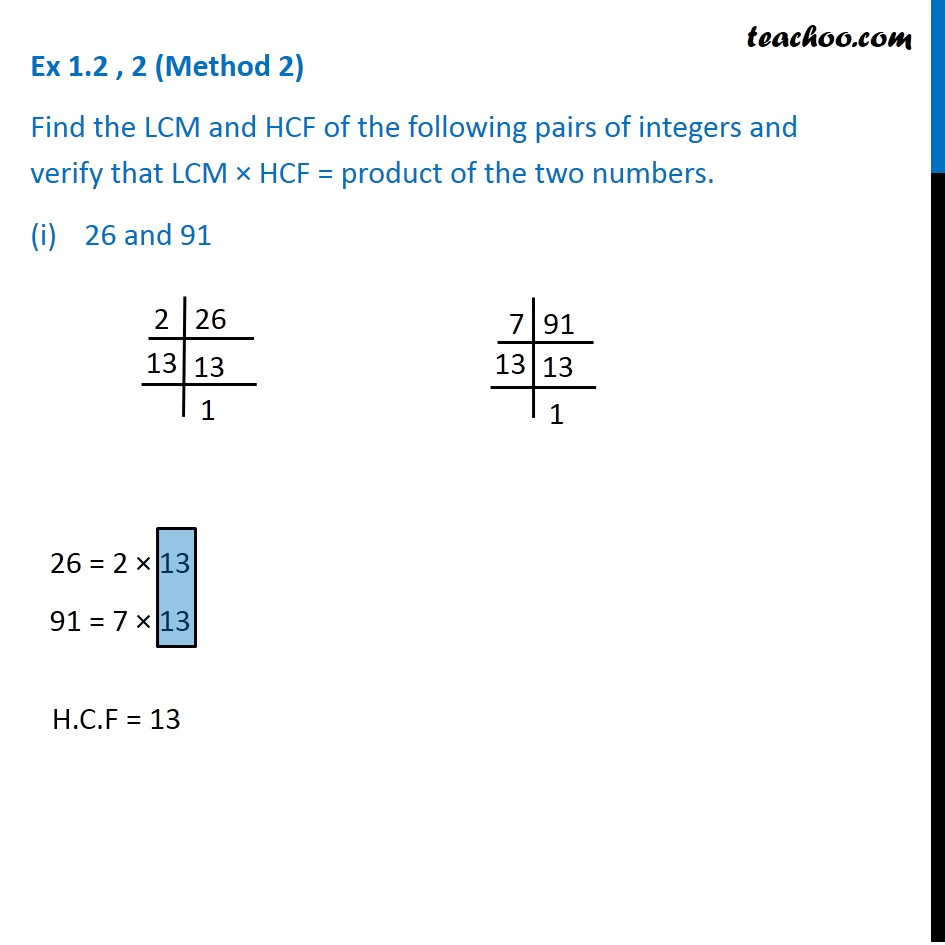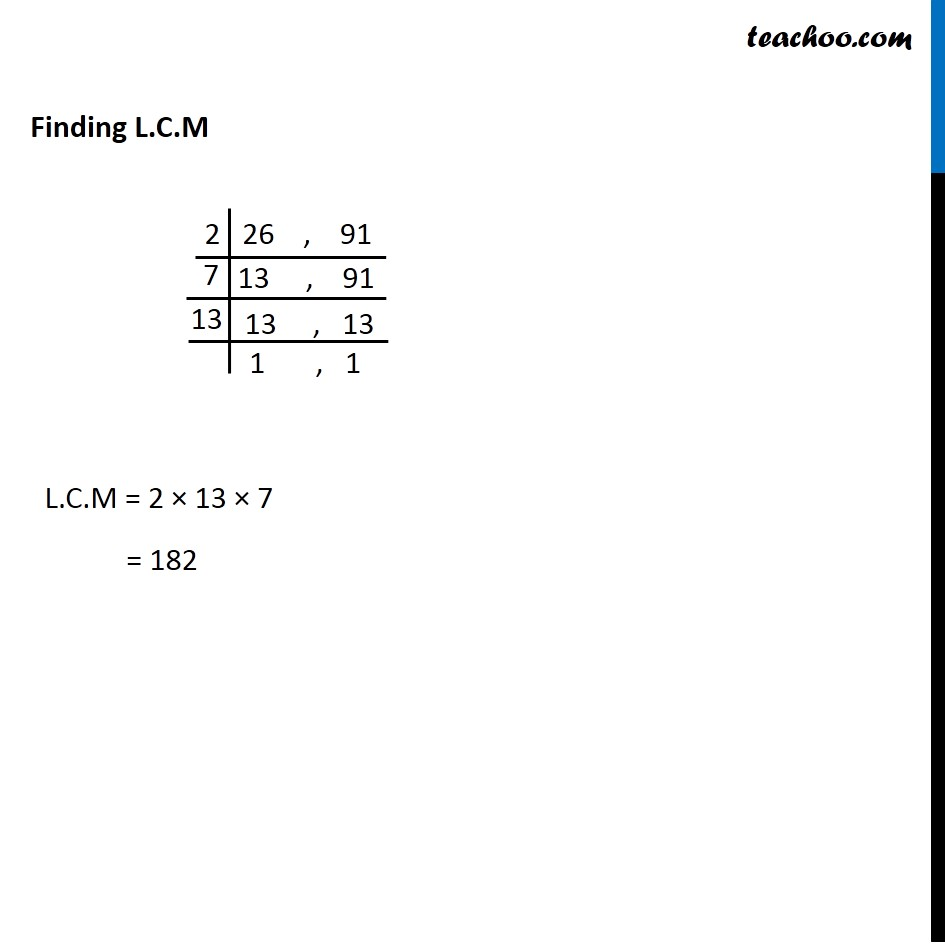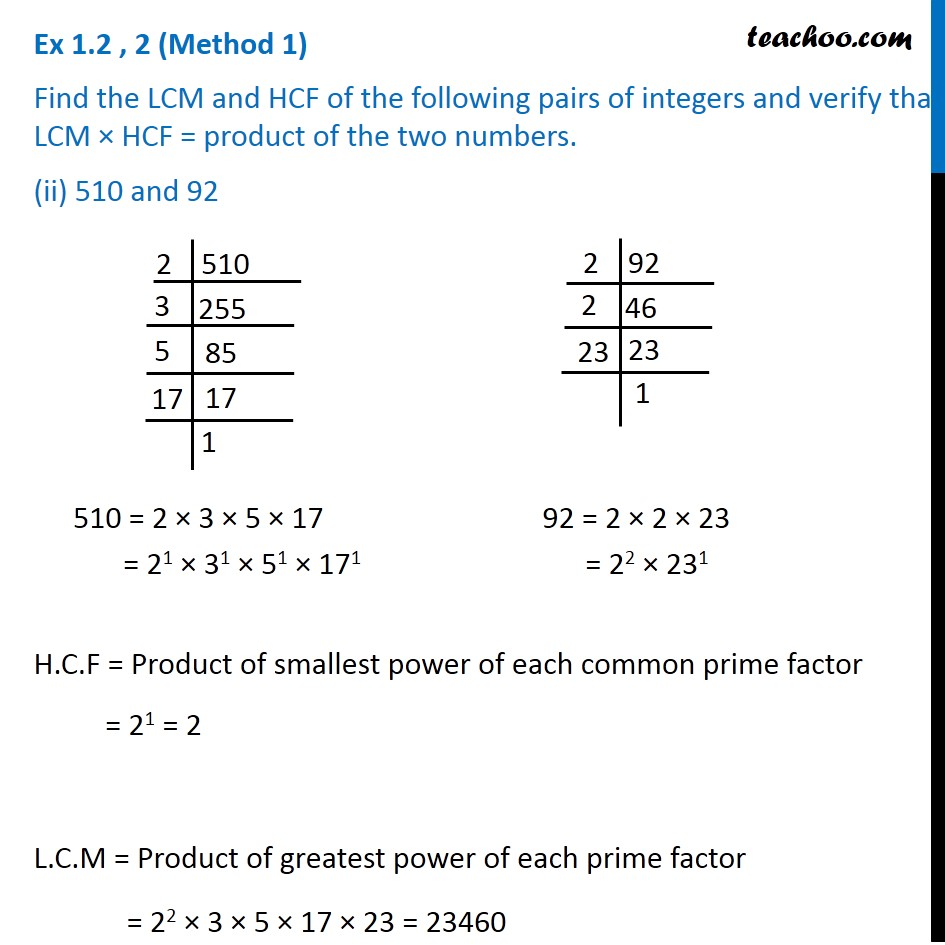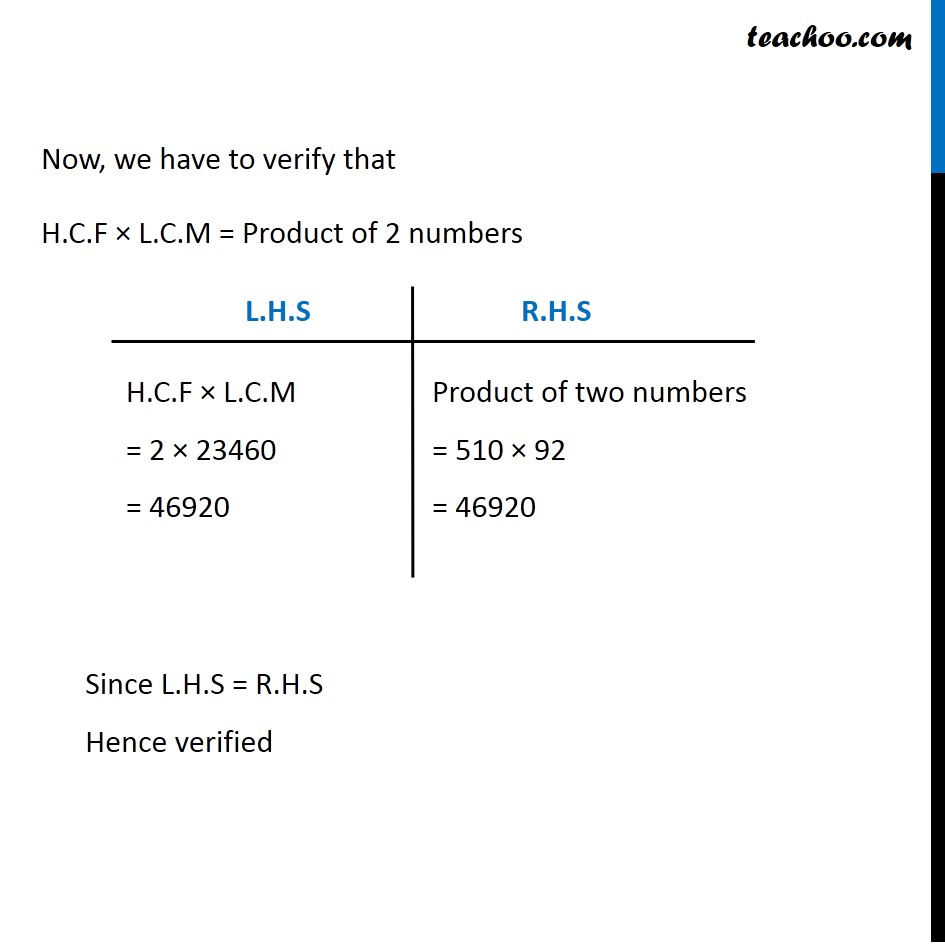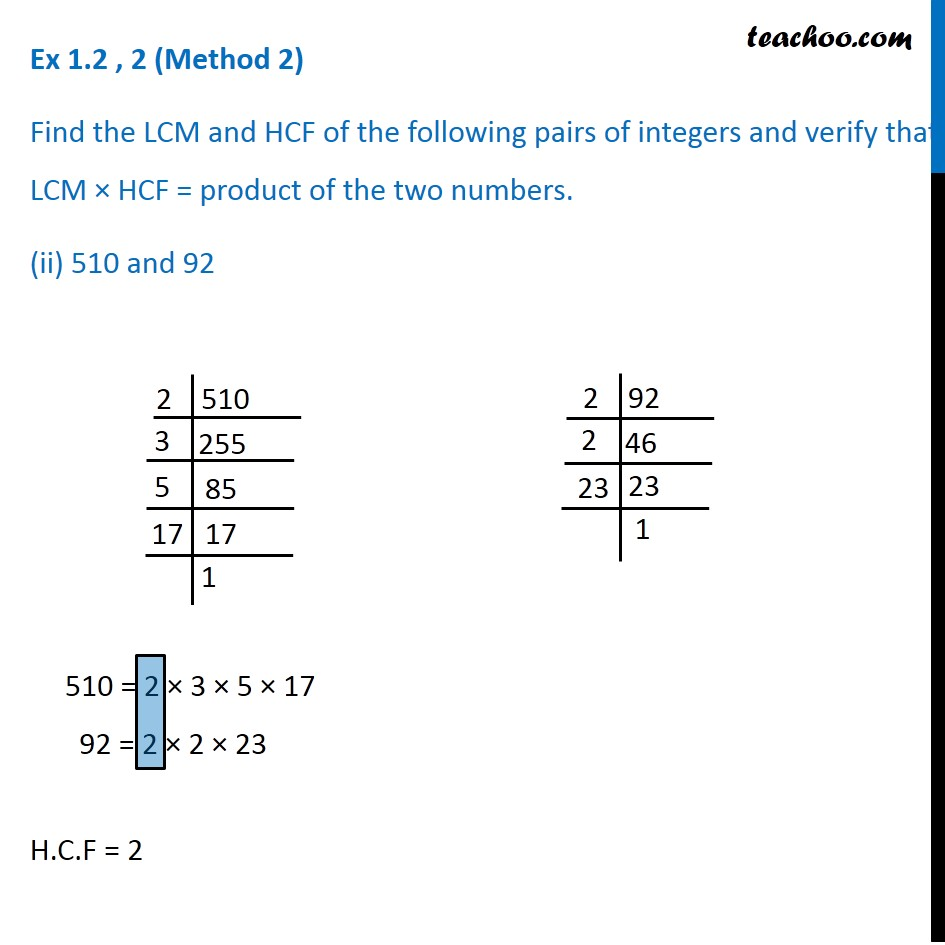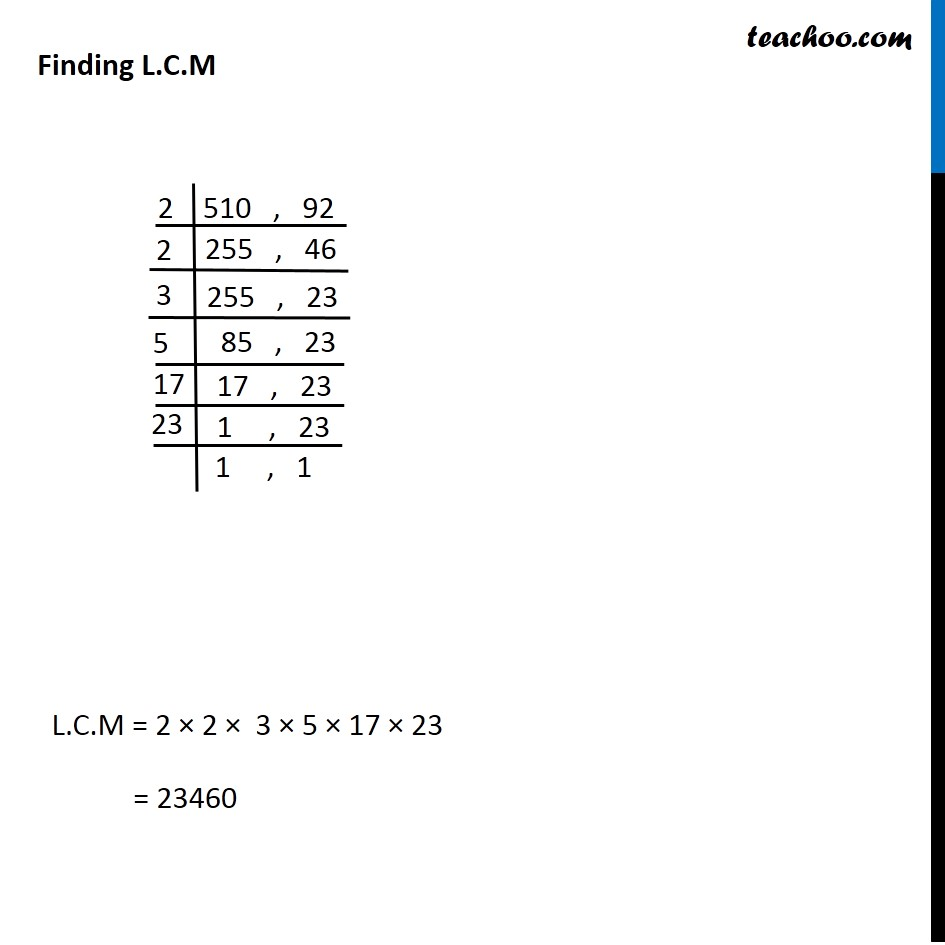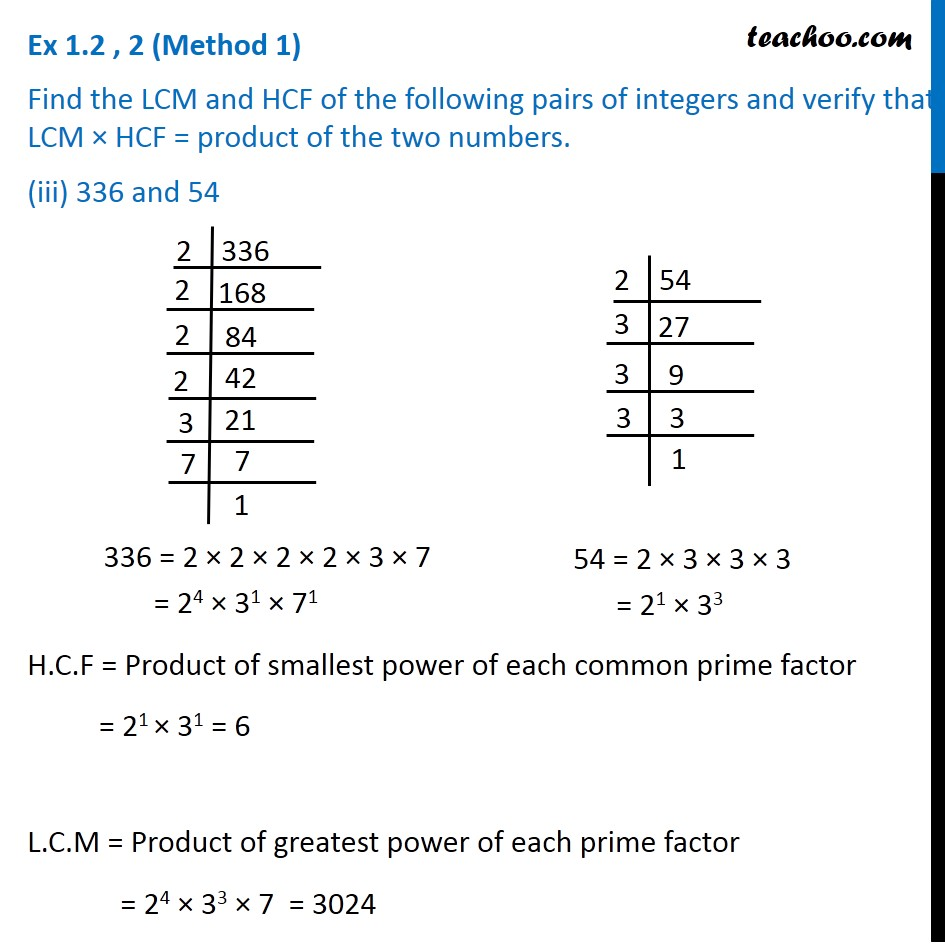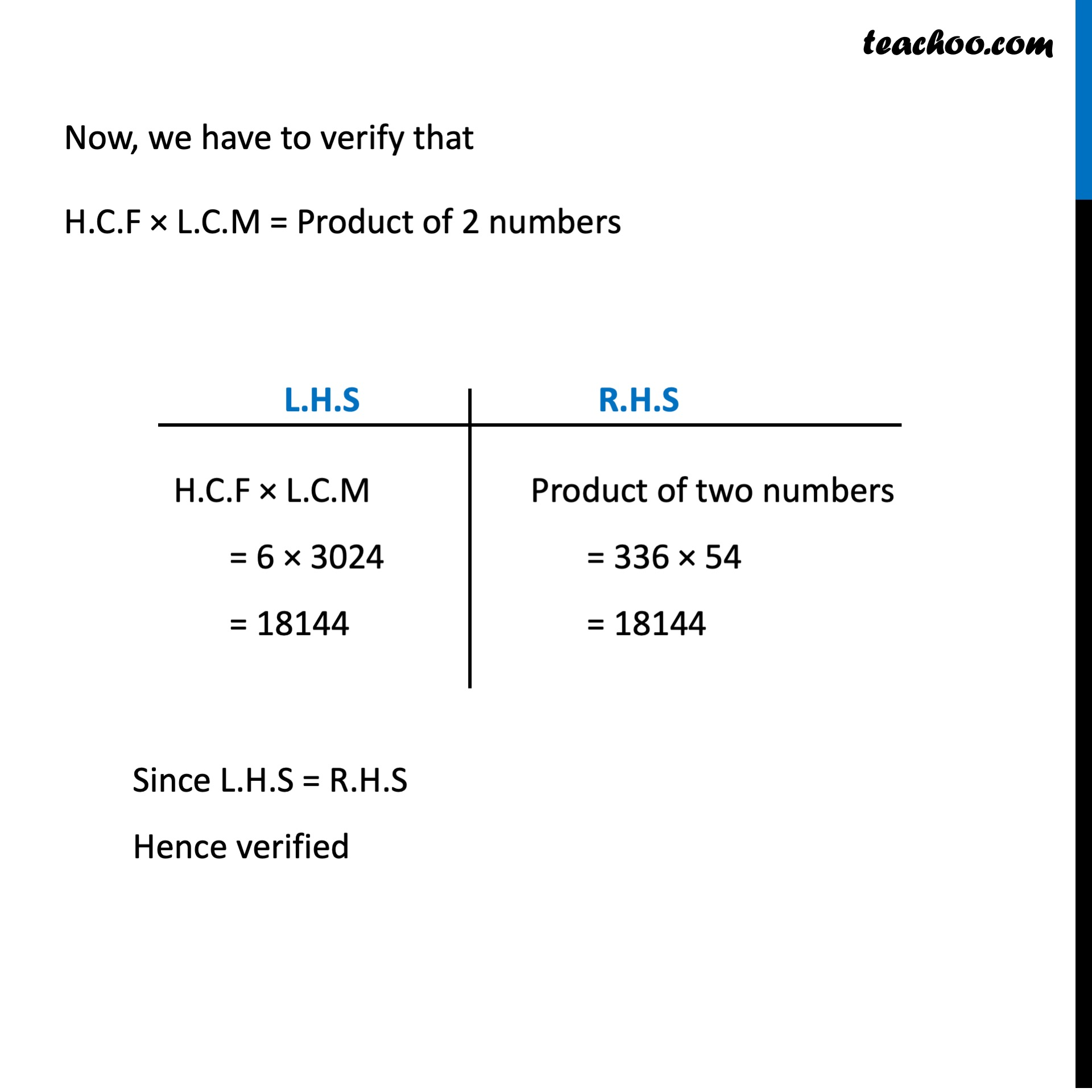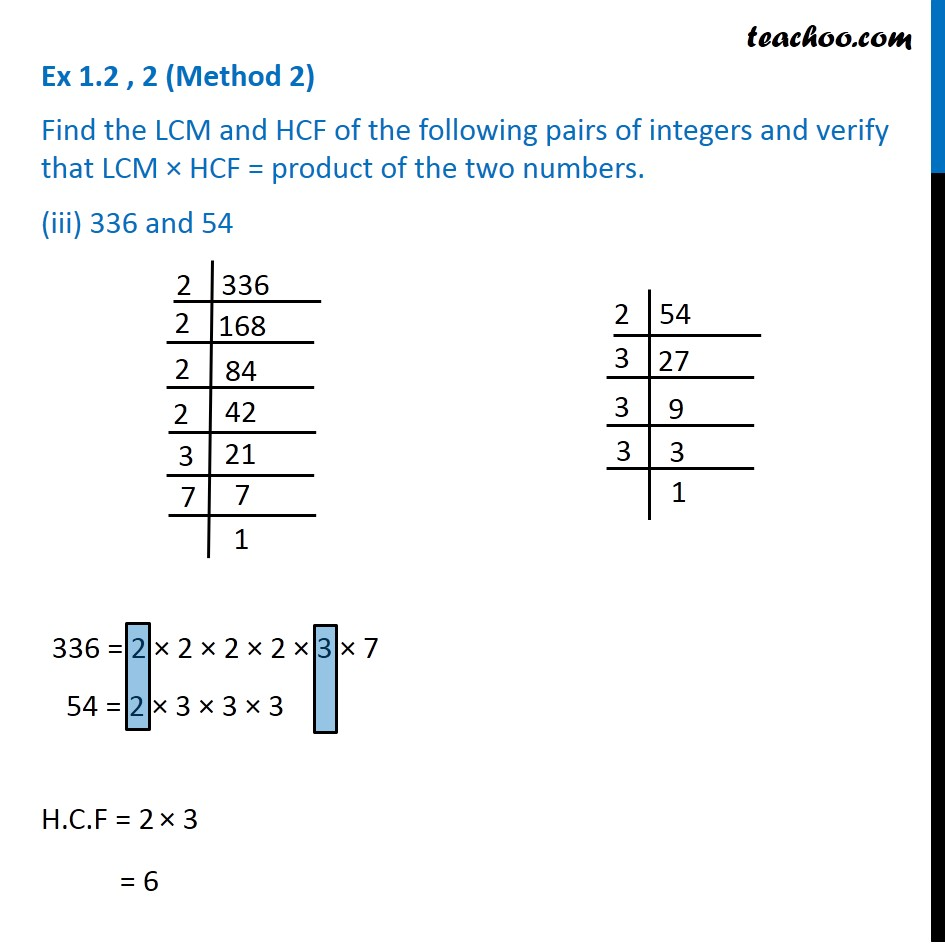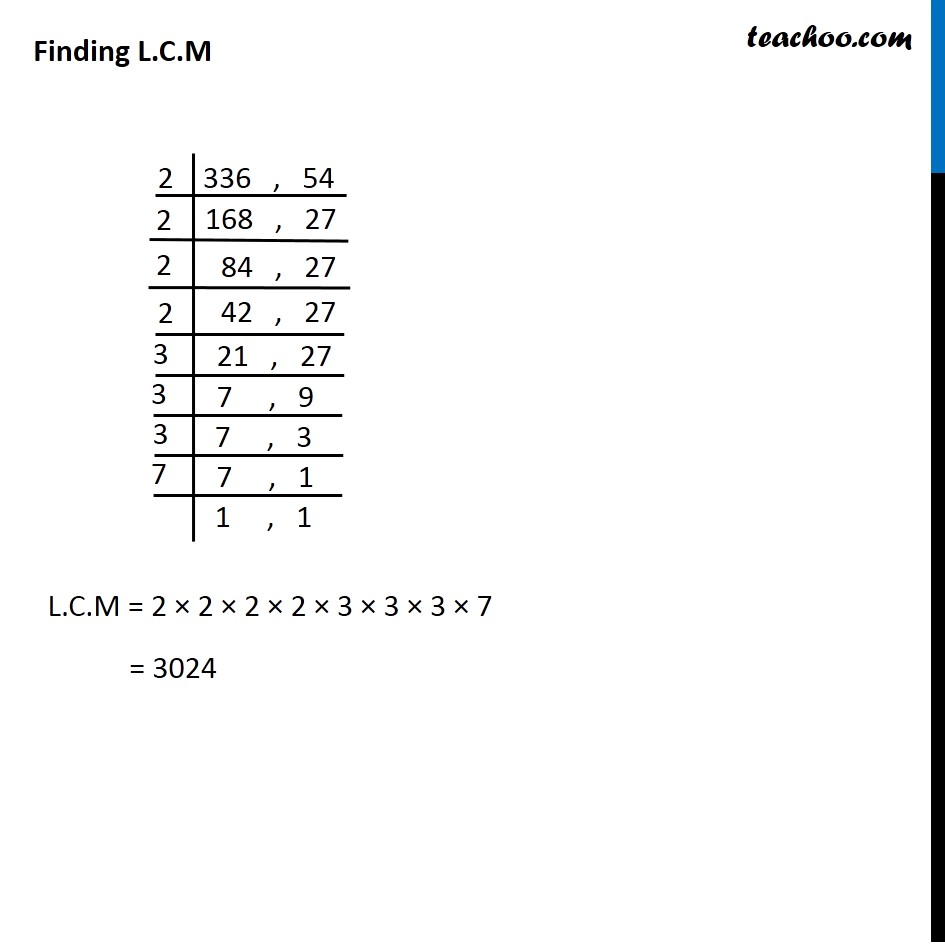1. Chapter 1 Class 10 Real Numbers
2. Serial order wise
3. Ex 1.2

Transcript

Ex 1.2 , 2 (Method 1) Find the LCM and HCF of the following pairs of integers and verify that LCM HCF = product of the two numbers. 26 and 91 H.C.F = Product of smallest power of each common prime factor = 13 L.C.M = Product of greatest power of each prime factor = 2 13 7 = 182 Now, we have to verify that H.C.F L.C.M = Product of 2 numbers Ex 1.2 , 2 (Method 2) Find the LCM and HCF of the following pairs of integers and verify that LCM HCF = product of the two numbers. 26 and 91 H.C.F = 13 Finding L.C.M L.C.M = 2 13 7 = 182 Now, we have to verify that H.C.F L.C.M = Product of 2 numbers Ex 1.2 , 2 (Method 1) Find the LCM and HCF of the following pairs of integers and verify that LCM HCF = product of the two numbers. (ii) 510 and 92 H.C.F = Product of smallest power of each common prime factor = 21 = 2 L.C.M = Product of greatest power of each prime factor = 22 3 5 17 23 = 23460 510 = 2 3 5 17 = 21 31 51 171 92 = 2 2 23 = 22 231 Now, we have to verify that H.C.F L.C.M = Product of 2 numbers H.C.F L.C.M = 2 23460 = 46920 Product of two numbers = 510 92 = 46920 Ex 1.2 , 2 (Method 2) Find the LCM and HCF of the following pairs of integers and verify that LCM HCF = product of the two numbers. (ii) 510 and 92 H.C.F = 2 510 = 2 3 5 17 92 = 2 2 23 Finding L.C.M L.C.M = 2 2 3 5 17 23 = 23460 Now, we have to verify that H.C.F L.C.M = Product of 2 numbers Since L.H.S = R.H.S Hence verified H.C.F L.C.M = 2 23460 = 46920 Product of two numbers = 510 92 = 46920

Ex 1.2